HOME: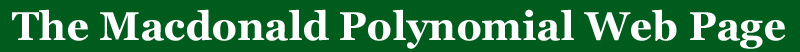BACK: More qt-analogs

# The number of rooted forests

 (n+1) n-1 XXX

The hilbert series for the diagonal harmonics is conjectured to q,t count the number of rooted forests of trees with labeled verticies.  These numbers arise naturally from an operator on symmetric functions that has the Macdonald polynomials as eigenfunctions.

Below is a table of these numbers for n between 2 and 6.  The total degree of these polynomials is n(n-1)/2.  It is conjectured that they have non-negative integer coefficients as polynomials in q and t.  Next to the tables 2 through 5 we have placed an image of forests of trees and next to each forest is the number of ways of labeling the forest and giving each tree a root (therefore the sum of these numbers will be (n+1)n-1).

These tables were taken from a much larger set that is available in postscript/LaTeX form available on the web page of Mark Haiman.  You will also find there the Frobenius series of the diagonal harmonics up to n=6.

 n=2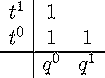n=3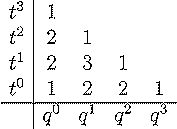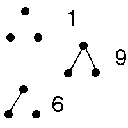n=4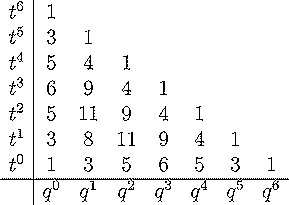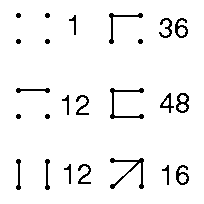n=5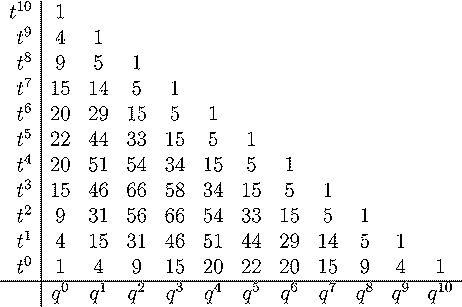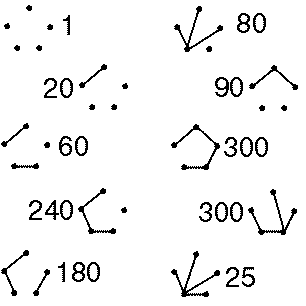n=6